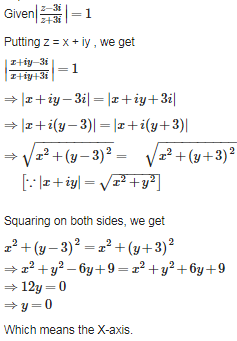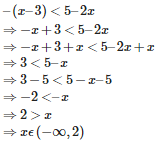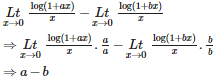# CBSE 11TH MATHEMATICS - Online Test

Q1. In a multiple choice question, there are 4 alternatives, of which one or more are correct. The number of ways in which a candidate can attempt this question is
Explaination / Solution:

Since given there are four alternatives in which one or more are correct,we have to consider the following four cases

1 correct answer can be chosen in 4C1 ways = 4 ways

2 correct answer can be chosen in 4C2 ways= 6 ways

3 correct answers can be chosen in  4C3ways  = 4 ways

4 correct answers can be chosen in4C4 ways = 1 way

Hence the totalnumber of ways = 4 + 6 + 4+ 1=15ways

Q2. If the line 2x – y +  = 0 is a diameter of the circle  then  =
Explaination / Solution:

Equation of circle is

Applying completing the square method

Comparing the above equation with   we get center as (-3,3) and radius as    .

As centre of the circlre lies on diameter , it will satisfy the equation of diameter, so on putting (-3,3) in equation of diameter we get

=>

=>

Q3. The domain of the function √cosx-1
Explaination / Solution:

This function exists only if cosx−1≥0 ⇒cosx≥1 ⇒cosx>1 OR cosx=1 since maximum value of cosine function is 1 so, cos x >1 is not possible so, cosx=1 cosx=cos2nπ ⇒x=2nπ(n∈I)
Q4. In a triangle ABC, tan $,$ then tan  is equal to
Explaination / Solution:Q5. The distance of the point ( 3, 4, 5) from X- axis is
Explaination / Solution:

The distance of the point ( 3, 4, 5) from X- axis is

let L be the foot of perpendicular from the point ( 3, 4, 5) to X axis ,then coordinate of L will be (3,0,0)       [ because on X axis y and z coordinate are zero]

then distance of the point ( 3, 4, 5) from X- axis i.e. from L (3,0,0) is given by

Q6. If A and B are two sets, then {tex}A \cap (A \cup B)'{/tex} is equal to
Explaination / Solution:

{tex}A \cap (A \cup B)' = \phi {/tex} since {tex}(A \cup B)'{/tex} Set represent the element which are not belongs to it,so there is no common element with the set A.So the answer is null set
Q7. The complex numbers z = x + iy which satisfy the equation  lie on
Explaination / Solution:Q8.

If n is a rational number, which is not a whole number, then the number of terms in the expansion of  is

Explaination / Solution:Q9. Identify the solution set for –( x – 3 ) < 5 – 2x
Explaination / Solution:Q10.  is equal to
Explaination / Solution: